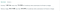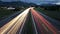Noob v. LeetCode, episode 6

In my ongoing quest to do battle with the ‘Easy’ algorithm questions in LeetCode, I came up against the challenge of converting Roman Numerals into integers.

Examples from LeetCode:

Fortunately, I was in the middle of a long drive home with my husband, who is software engineer and used to these kinds of puzzles. The first thing we discussed was how to know when to subtract a Roman numeral from the one after it.

I was thinking on the lines of, if it’s an “I” and it’s followed by a “V”, we’ll subtract. Oh right, and if it’s an “I” before “X”, we’ll also subtract. And so on and so on and oh my gosh, that was going to be a long list of rules for when to subtract.

My partner in crime had a better, more abstract approach: if the Roman numeral we’re looking at is the same or equal to the next one, we can add it to the total. If it’s less than the next one, we will subtract it from the next one, and then add that result to the total.

If you already see the shortcut, good for you. If not, bear with me!

Breaking it down

Once we got home, I took out my computer and broke the problem down into smaller problems. I had to figure out how to:

1. Convert individual Roman numerals into their corresponding numeric value
2. Set rules for when to add and subtract
3. Return a total

Step 1. Convert individual Roman numerals to numeric values

I knew that I wanted “I” to equal 1, “V” to equal 5, etc.

So I tried a long list of constants:

const “I” = 1
const “V” = 5
etc.

The problem is, “I” is a string, not a variable. If you want to convert it into a variable, there is a lot of conflicting advice on how to do that. I wanted to keep things as simple as possible. After many dead ends, I finally Googled to see how other people do this.

I glimpsed this and decided to see how far I could run with it, without reading the explanation:

So I set up my own arrays:

const romanNumerals = ["M", "D", "C", "L", "X", "V", "I"];
const integers = [1000, 500, 100, 50, 10, 5, 1];

The test case input for this puzzle was “III”. I knew I was going to want to look at each one of those “I”s individually, so I split the string into an array:

let array = s.split('')

This gave me: ["I", "I", "I"]

IndexOf()

Now I had to examine each element, find its index in the romanNumerals array, and then translate it to the numeric value stored at the same index in the integers array. This is trivial (fellow noobs: that means easy to do; there is no negative connotation as there is in English!) with JavaScript’s indexOf().

So, if I’m looking at “I”, I can get the index of that string with romanNumerals.indexOf(“I”), which by the way is 6.

To convert to a numberic value, I just need to call out the element at the same index in the integers array:integers,

except I don’t really need to know that the index is 6 — all I need to know is that it is the same as the romanNumeral’s index in the romanNumerals array. So I can use:

integers[romanNumerals.indexOf(“I”)] which gives me: 1

Now I need to do that for every character in the Roman Numeral string; and ( need to add or subtract the result to a counter, called total.

Revised Step 2: Figure how to add each numeric value to a total.

Instead of setting rules for when to add or subtract, I decided to first write a program that would simply add the value of each character to a total.

I started out with this:

//test value:
s = "III"
array = s.split('')//set a counter called 'total' with an initial value of zero:
let total = 0

for(let i = 0; i < array.length; i++){
total += integers[romanNumerals.indexOf(array[i])]
}

// => 3

Hurrah, my test case worked! At least I got this far…

Revised Step 3. Figure out when to add and when and how to subtract

Earlier, I had decided to add a value to my total if it was greater than the next value; otherwise I’d subtract it from the next value and add that result to my total.

So I wrote a way to compare the current value to the next value.

If the current element in my array is array[i] , then the next value is array[i+1].

If the numeric value of array[i]is integers[romanNumerals.indexOf(array[i])]

then the numeric value of array[i+1] is integers[romanNumerals.indexOf(array[i+1])]

So if we want to see if the current value is greater than or equal to the next value, we can write something like this:

if(integers[romanNumerals.indexOf(array[i])] >= integers[romanNumerals.indexOf(array[i+1])] ...//feeling dizzy??

That code felt like a house of mirrors! To make it easier for me to understand, I added a few variables:

let current;
let currentValue;
let next;
let nextValue;
for (let i=0; i < array.length; i++){
current = array[i];
currentValue = (integers[romanNumerals.indexOf(current)]);
next = array[i+1];
nextValue = (integers[romanNumerals.indexOf(next)]);
//...

That made it easier to write the next part:

if (currentValue >= nextValue ){
// add current value to total
} else if (currentValue < nextValue) {
//subtract current value from next value and add result to total ?
}

The problem with IX …

Our for loop is set up to process one element at a time, decide how to use it to change the total, and then move on to the next element. But in the ‘else if’ part of the code, we’re skipping ahead by dealing with two values at a time, array[i] and array[i+1].

For example. If we have “I” followed by “X”, we translate “I” to 1 and “X” to 10. Following our rule, since 1 is less than 10, we subtract 1 from 10 for a result of 9; then we add 9 to our total.

But in the next loop, our current element is the previous ‘next’ element, “X” with a value of 10. But we already factored that 10 into our total on the last loop. If we consider that same 10 again, we’ll throw off our total!

Down the Rabbit Hole

This presented an excellent opportunity to go deep down the rabbit hole. I was considering incrementing i by 2 inside that else statement when I realized …

I didn’t have to deal with two values at once. If an “I” preceded an “X”, (or a “C” preceded a “D” etc.) I could simply subtract its numeric value from the total, and then add the numeric value for the “following roman numeral” on the next loop.

Why was I trying to do it the other way in the first place? Because I was thinking way too literally: since “IX” in Roman Numerals is 9, I was thinking I had to parse those characters as a pair, instead of simply subtracting 1 and adding 10.

Revised Step 4: return the total

After my for loop, I returned the total. Here’s whole function at this point:

const romanToInt = function(s) {
const array = s.split('');
const romanNumerals = ["M", "D", "C", "L", "X", "V", "I"];
const integers = [1000, 500, 100, 50, 10, 5, 1];

let total = 0 ;
let current;
let currentValue;
let next;
let nextValue;
for (let i=0; i < array.length; i++){
current = array[i];
currentValue = (integers[romanNumerals.indexOf(current)]);
next = array[i+1];
nextValue = (integers[romanNumerals.indexOf(next)]);
if (currentValue >= nextValue ){
total += (currentValue);
} else if (currentValue < nextValue) {
total -= (currentValue);
} else if (currentValue && !nextValue) {
total += currentValue
}

}
}Not bad! we’re in that top 25% for runtime, though we could do better with memory usage.

Can we make this faster with a hash?

I also tried setting up my Roman Numeral and integer info as a hash instead of as two arrays:

const romanToInt = function(s) {
const array = s.split('');

const conversion = {"M": 1000, "D":500, "C":100, "L":50, "X":10, "V":5, "I":1}
// const romanNumerals = ["M", "D", "C", "L", "X", "V", "I"];
// const integers = [1000, 500, 100, 50, 10, 5, 1];

let total = 0 ;
let current;
let currentValue;
let next;
let nextValue;
for (let i=0; i < array.length; i++){
current = array[i];
currentValue = conversion[array[i]]
// currentValue = (integers[romanNumerals.indexOf(current)]);
next = array[i+1];
nextValue = conversion[array[i+1]]
// nextValue = (integers[romanNumerals.indexOf(next)]);
if (currentValue >= nextValue ){
total += (currentValue);
} else if (currentValue < nextValue) {
total -= (currentValue);
} else if (currentValue && !nextValue) {
total += currentValue
}

}
}

I’m not sure why, but it was actually slightly slower (which made its runtime rankings way worse); it also used slightly less memory, which made its memory rankings way better:

Major improvement

The biggest improvement came from cleaning up this part of the solution:

if (currentValue >= nextValue ){
total += (currentValue);
} else if (currentValue < nextValue) {
total -= (currentValue);
} else if (currentValue && !nextValue) {
total += currentValue
}

I had originally written it like so:

if (currentValue >= nextValue ){
total += (currentValue);
} else if (currentValue < nextValue) {
total -= (currentValue);
}

but it didn’t work, because of the edge case, when currentValue is the last value in the array. When that happens, there is no nextValue to compare to, so the code skipped evaluating the last value. That’s why I added :

else if (currentValue && !nextValue) {
total += currentValue
}

i.e. if there is no next value, go ahead and add current value to the total.

BUT … that slowed the code down a lot. Looking through the other solutions on LeetCode, I noticed this one from hadleyac (honest, I didn’t look at it until I got this far writing the code on my own! it literally popped up a few minutes ago …), which took the same approach, but handled the if statement in a cleaner way. So I refactored:

if (currentValue < nextValue ){
total -= (currentValue);
} else {
total += (currentValue);
}

This covers the case where the currentValue is also the last value.

All together now:

const romanToInt = function(s) {
const array = s.split('');
const romanNumerals = ["M", "D", "C", "L", "X", "V", "I"];
const integers = [1000, 500, 100, 50, 10, 5, 1];

let total = 0;
let current;
let currentValue;
let next;
let nextValue;

for (let i=0; i < array.length; i++){
current = array[i];
currentValue = (integers[romanNumerals.indexOf(current)]);

next = array[i+1];
nextValue = (integers[romanNumerals.indexOf(next)]);
if (currentValue < nextValue ){
total -= (currentValue);
} else {
total += (currentValue);
}
}

This final change made a BIG difference in runTime:

Woot!no speed limit on the autobahn …

Next: Algorithms 101, episode 7: House Robber in JavaScript

In case you missed it: Algorithms 101, episode 5: Goat Latin in JavaScript

Written by

JavaScript in Plain English

Learn the web's most important programming language.

Welcome to a place where words matter. On Medium, smart voices and original ideas take center stage - with no ads in sight. Watch
Follow all the topics you care about, and we’ll deliver the best stories for you to your homepage and inbox. Explore
Get unlimited access to the best stories on Medium — and support writers while you’re at it. Just \$5/month. Upgrade